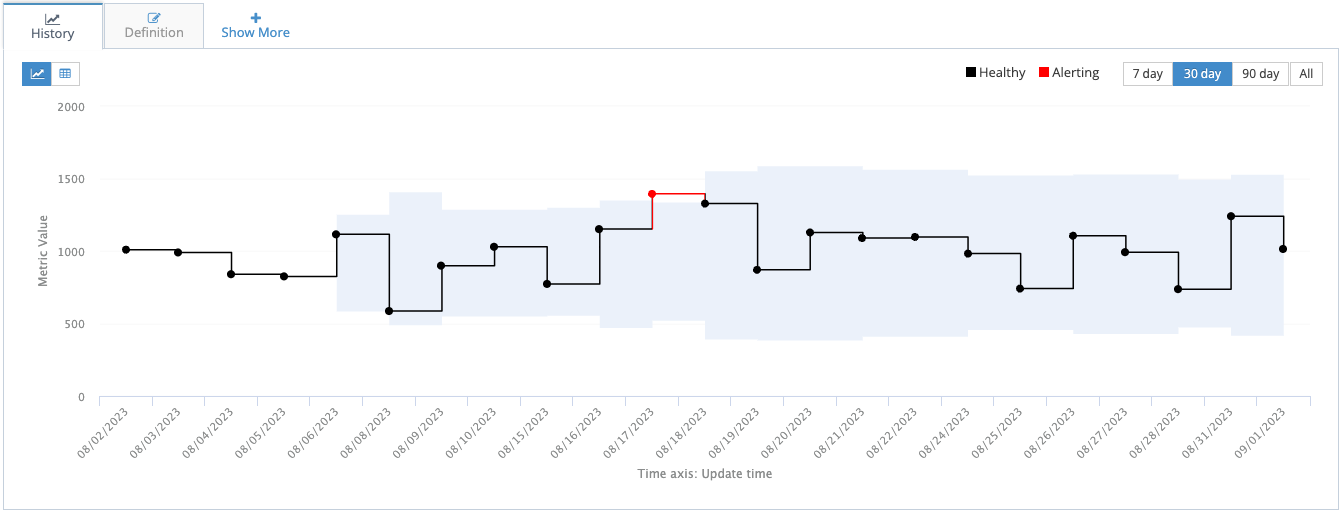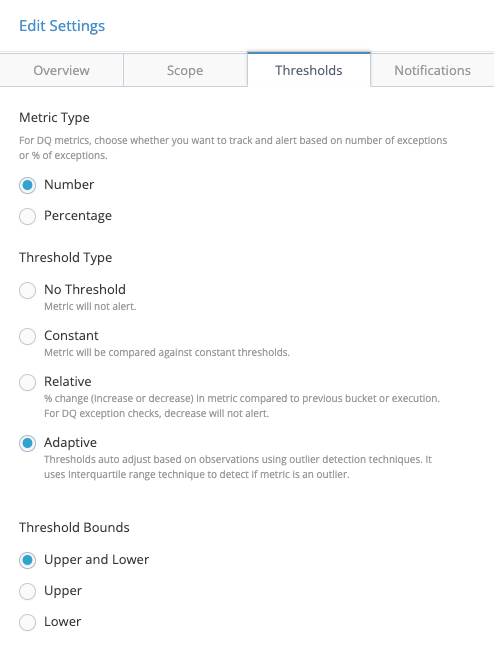# Adaptive Thresholds in Data Quality

## When to use Adaptive Thresholds

Classic data quality (DQ) rules define a test that must be met for data to be considered valid. This could be a simple not-null test or a cross reference integrity check or a more complex calculation. Typically the acceptable maximum threshold for this test would be zero. Invalid values are not acceptable.

But it turns out there are many real-world reasons to allow more flexible thresholds. One reason is that data is known to have a baseline number of invalid records. This is not desired, but it's expected. And in practice the team monitoring data quality doesn't want to receive a notification every day that there are invalid records--since that might well be every single day. But they would certainly be interested to get a notification if the number of invalid records spikes one day.

An even more common use case for wanting adaptive thresholds is for data observability tests. In many situations the number of records arriving each day should be roughly the same. A small variation is expected, but a large variation could indicate a problem. But how can we quantify "small variation" and "large variation"? This is where adaptive thresholds can help.

## How Adaptive Thresholds are Calculated

Adaptive thresholds in DvSum DQ are set using the common statistical technique called Interquartile Range (IQR). In this method, we first calculate the 25th percentile and 75th percentile for past values. These values are referred to as the 1st quartile (Q1) and 3rd quartile (Q3). The IQR is simply the difference between Q3 and Q1. We define outliers as values which are more than 1.5 IQRs below Q1 or more than 1.5 IQRs above Q3.

### Example

An example of the adaptive thresholds in action makes them clear. Consider the following values for the number of records loaded daily by a data pipeline.You can also toggle to display the values in a grid. Here are those values:

1009, 990, 840, 825, 1115, 586, 899, 1029, 773, 1151, 1394, 1327, 870, 1128, 1089, 1097, 982, 741, 1105, 992, 737, 1240, 1014.

The first 4 data points have no thresholds displayed. This is because the IQR threshold calculation requires a minimum of 4 previous data points to be meaningful. The thresholds for the 5th data point are calculated as follows.

`The 4 previous data points: 1009, 990, 840, 825Q1: the median of 825 and 840 = 832.5Q3: the median of 990 and 1009 = 999.5IQR: Q3 - Q1 = 167IQR * 1.5 = 250.5Upper Threshold: 999.5 + 250.5 = 1250Lower Threshold: 832.5 - 250.5 = 582`

The same logic applies in identifying the data on Aug 17 as an outlier. The previous data points (1009, 990, 840, 825, 1115, 586, 899, 1029, 773, 1151) yield a Q1 value of 825 and a Q3 value of 1029. Those correspond to threshold values of 519 and 1,335.

### Why IQR * 1.5?

The intuitive answer is that if the data is truly following a normal distribution and we're only seeing random noise in the results, then setting thresholds like this are expected to generate alerts only very rarely. The data quality team won't get too many notifications. But how many?

The complete details of the mathematical calculation are beyond the scope of this article. But setting the bounds at Q1 - 1.5*IQR and Q3 + 1.5*IQR will correspond to setting the bounds at μ ± 2.7σ. With these bounds slightly fewer than 1% of measurements from a normal distribution will generate alerts.

Stated in a less-precise but more business-oriented manner, if you get an alert then it's quite likely that something went wrong. Maybe a file got loaded twice, or the underlying data has truly changed. You should investigate. But of course it's possible (about a 0.7% chance) that this is just a random fluctuation in the data.

## How to set Adaptive Thresholds

Prerequisite: you have already created a DQ rule. Pipeline checks like COUNT and METRIC are the most common types to use adaptive thresholds. For more details about how to define DQ rules, refer to the DQ Rule Details page.

Edit the rule, then navigate to the tab "Thresholds".

Thresholds for DQ rules are set in this dialog in DvSum Data Quality: